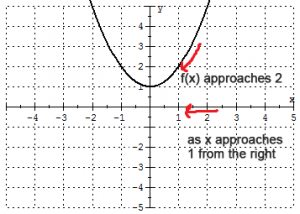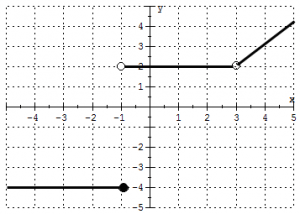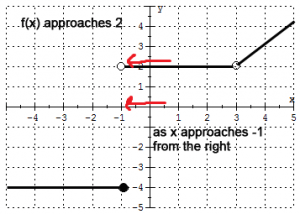# Finding Limits Using a Graph

## What is a limit?

Calculus involves a major shift in perspective and one of the first shifts happens as you start learning limits. When I talk about the limit of a function $$f(x)$$ as $$x$$ approaches some value, I am not saying “what is $$f(x)$$ at this value” like I might in algebra! Instead, I am interested in what is happening to $$f(x)$$ when $$x$$ is close to this value.

## Notation

If we are interested in what is happening to the function $$f(x)$$ as $$x$$ gets close to some value $$c$$ from the right, we write:

$$\lim_{x \to c^+} f(x)$$
This is called the right handed limit.

If we are interested in what is happening to the function $$f(x)$$ as $$x$$ gets close to some value $$c$$ from the left, we write:

$$\lim_{x \to c^-} f(x)$$
This is called the left handed limit.

## Example

Now for an actual example! I have graphed the function $$f(x)=x^2+1$$ below. We are going to find two limits: The limit of $$f(x)$$ as $$x$$ approaches 1 from the right and the limit as $$x$$ approaches 1 from the left. Remember: I don’t care what is happening when $$x=1$$, I only care about what is happening what $$x$$ is close to 1!From the picture above, I can see that $$\lim_{x \to 1^-} f(x)=2$$ and $$\lim_{x \to 1^+} f(x)=2$$. When both the right hand and left hand limits exist (there will be a different discussion about when limits don’t exist) and equal, then we say the two sided limit equals that value (when people say “the limit” they usually mean the two sided limit). In this case $$\lim_{x \to 1} f(x)=2$$. If the left and right hand limits were different numbers, we would say the two sided limit doesn’t exist.

Did you notice that here it is also true that $$f(1)=2$$? This does not always have to be the case! Take a look at this piece-wise defined function (that means there is a different definition for the function for different parts of the domain).Notice the closed and open circles. The open circle at a y-value means that is not a value of the function when you plug in $$x$$. For example, $$f(-1) = -4$$ since that is where the solid circle is. Additionally, $$f(3)$$ is undefined since there is no solid circle at $$x=3$$. But what about the limits?From the picture above, we see that $$\lim_{x \to 3^-} f(x)=2$$ and $$\lim_{x \to 3^+} f(x)=2$$ therefore $$\lim_{x \to 3} f(x)=2$$ even though $$f(3)$$ is undefined! Again, it doesn’t matter what is happening when $$x=3$$ only what is happening near that value!However, $$\lim_{x \to -1^-} f(x)=-4$$ and $$\lim_{x \to -1^+} f(x)=2$$. Therefore, $$\lim_{x \to -1} f(x)$$ does not exist, even though $$f(-1)=-4$$. I don’t have to say it again do I? (the whole thing about we are looking near x not at x… there I said it…)

As you continue to study limits, the plan is to develop ways to find limits without using the graph, but being able to find a limit this way can give you a much better understanding of exactly what a limit is, even if you aren’t using the formal definition.